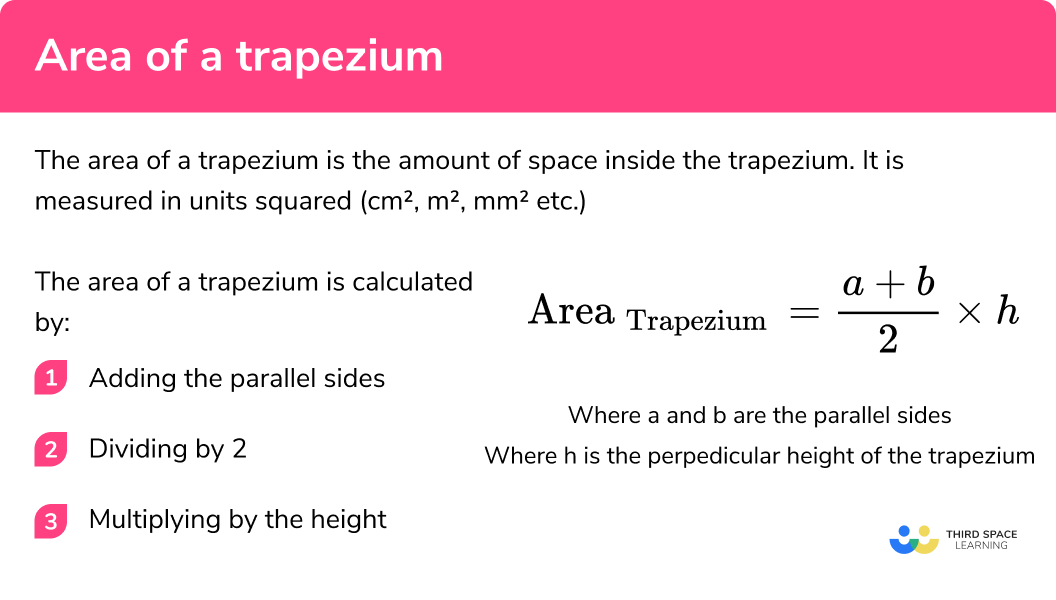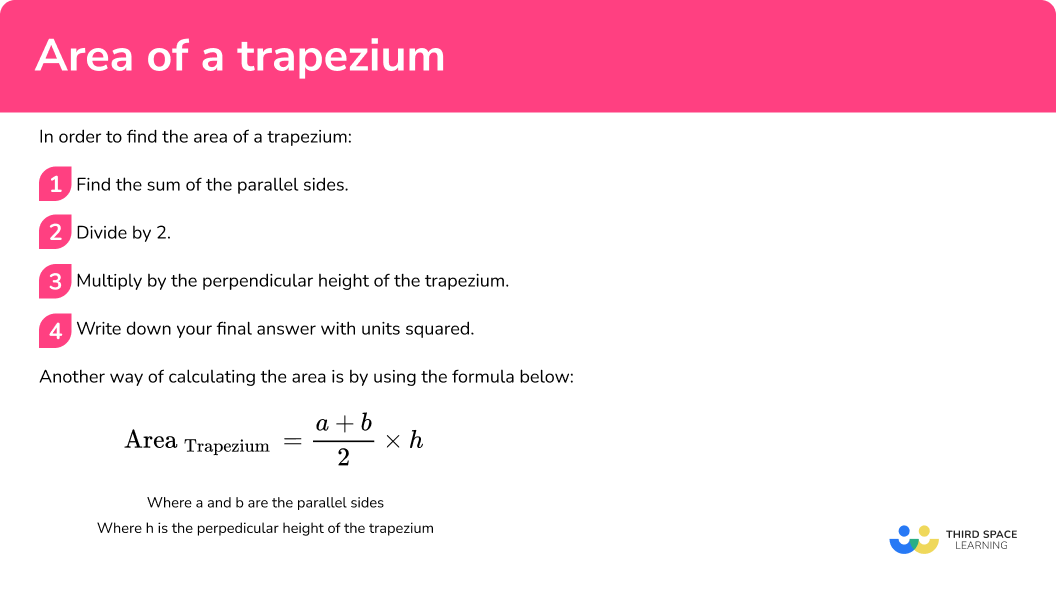GCSE Maths Geometry and Measure Area

Area Of A Trapezium

# Area Of A Trapezium

Here we will learn about finding the area of a trapezium, including compound area questions, questions with missing side lengths and questions involving unit conversion.

There are also area of a trapeziumworksheets based on Edexcel, AQA and OCR exam questions, along with further guidance on where to go next if you’re still stuck.

## What is the area of a trapezium?

The area of a trapezium is the amount of space inside the trapezium. It is measured in units squared ( cm^{2}, m^{2}, mm^{2} etc.)

A trapezium (also known as a trapezoid) is a quadrilateral with exactly one pair of parallel sides. The parallel sides are indicated using small arrows.

The plural of trapezium is trapezia or trapeziums.

An isosceles trapezium is a trapezium where the base angles are equal and therefore the left and right side lengths are also equal.

The area of a trapezium is calculated by:

2. Dividing by 2
3. Multiplying by the height.

This can also be summarised with the following formula:

\text { Area }_{\text {Trapezium }}=\frac{a+b}{2} \times h

Where a and b are the parallel sides

Where h is the perpendicular height of the trapezium

### What is the area of a trapezium?## How to find the area of a trapezium

In order to find the area of a trapezium

1. Find the sum of the parallel sides.
2. Divide by 2 .
3. Multiply by the perpendicular height of the trapezium.

Another way of calculating the area is by using the formula below:

\text { Area }_{\text {Trapezium }}=\frac{a+b}{2} \times h

Where a and b are the parallel sides

Where h is equal to the perpendicular height of the trapezium

### How to find the area of a trapezium## Related lessons on area

Area of a trapezium is part of our series of lessons to support revision on area. You may find it helpful to start with the main area lesson for a summary of what to expect, or use the step by step guides below for further detail on individual topics. Other lessons in this series include:

## Area of a trapezium examples

### Example 1: finding area given the parallel side lengths and height

Find the area of the trapezium below:

1. Find the sum of the parallel sides.

4+6=10

2Divide by 2.

10\div2=5

3Multiply by the perpendicular height of the trapezium.

In this case the perpendicular height is 3 .

5\times3=15

\text { Area }=15 \mathrm{~m}^{2}

### Example 2: finding the area of a trapezium requiring converting units

Find the area of the trapezium below:

Find the sum of the parallel sides.

Divide by 2.

Multiply by the perpendicular height of the trapezium.

### Example 3: worded question

Sarah is looking to design the flower bed below at the front of her house. She would like to cover the entire flower bed with rose bushes. Each bush needs 1.5m^{2} to allow the roots to spread out evenly. What is the maximum number of rose bushes she can plant on her flower bed?

Find the sum of the parallel sides.

Divide by 2.

Multiply by the perpendicular height of the trapezium.

### Example 4: area of compound shapes

Find the area of the shaded region below:

Find the sum of the parallel sides.

Divide by 2.

Multiply by the perpendicular height of the trapezium.

### Example 5: calculating height given the area

The area of the trapezium below is 128m^{2} . Calculate the value of h in the trapezium below:

Rearrange the area of a trapezium formula

Substitute in the known values

### Example 6: calculating base length given the area

The area of a trapezium is 322 square millimetres and its height is 14mm . If the length of one of the parallel sides is 27mm , what is the length of the other parallel side?

Let’s start out by sketching out a trapezium using what is given in the question:

Rearrange the area of a trapezium formula

Substitute in the known values

### Common misconceptions

• Using incorrect units for the answer

A common error is to forget to include squared units when asked to calculate area.

• Forgetting to convert measures to a common unit

Before calculating the area of a trapezium, pupils must look at the units given in the question. If different units are given e.g. length = 4m and width = 3cm pupils must convert them either both to cm or both to m .

• Using length of the non-parallel sides when calculating area and not the height

Sometimes in a question we are given additional lengths which are not needed in our calculations. Sometimes we are given the length of the non-parallel sides of the trapezium. We must be careful to not mistake these measurements for height.

### Practice area of a trapezium questions

20.5m^{2}36m^{2}67.5m^{2}47.5m^{2}Add the parallel sides 9 and 7 together. Divide the sum by 2 . Times by the perpendicular height of 4.5 .

2. Find the area of the trapezium below:5085cm^{2}5085m^{2}2055.6cm^{2}2055.6m^{2}Convert 0.68m into cm . Add the 45cm and 68cm together. Divide by 2 . Multiply by 90 .

3. Sally is looking to design the garden below at the back of her house. She would like to cover the entire garden with grass. An individual carpet of grass covers an area of 4 square metres. How many rolls of grass will be needed to cover the entire garden?42.51011165Add the parallel sides 11 and 6 together. Divide the sum by 2 . Times by the perpendicular height of 5 . Divide by 4 .

4. Find the area of the shaded region below:224m^{2}84.5m^{2}44m^{2}55m^{2}Find the area of the rectangle by multiplying its length times width. (5 x 11) . Split the hexagon in half and find the area of each trapezium. To do this add the parallel sides ( 11 and 15 ), divide by 2 , and multiply by the perpendicular height which in this case is 6.5 . As there are two congruent trapeziums, multiply the area of the trapezium by 2 and add on the area of the rectangle to get the compound area.

5. The area of the trapezium below is 68m^{2} . Calculate the value of h in the trapezium below:8.5m^{2}60m^{2}16m8.5mStart with the area of a trapezium formula \text { Area }_{\text {Trapezium }}=\frac{a+b}{2} \times h and rearrange to get the formula h=\frac{2 A}{(a+b)} . Substitute the values of the area and the parallel sides and solve for the height.

6. Calculate the value of a in the trapezium below:55mm13mm45mm14mmStart with the area of a trapezium formula \text { Area }_{\text {Trapezium }}=\frac{a+b}{2} \times h and rearrange to get the formula a=\frac{2 A}{h}-b . Make sure to isolate a by moving the other variables to the other side of the equal sign and changing to the opposite sign. Substitute the known values and solve for the length of the parallel side.

### Area of a trapezium GCSE questions

1. Find the area of the trapezium below.(3 marks)

Finding sum of parallel sides (12+18=30)

(1)

Dividing by 2 or multiplying by height

(1)

75m^{2} (cao with units)

(1)

2. (a) Calculate the value of x in the trapezium below.(b) The above trapezium is enlarged by a scale factor of 2 . What is the area of the new trapezium?

(7 marks)

(a)
Evidence of adding the parallel sides 11+17 = 28

(1)

Dividing by 2

(1)

70\div14 seen

(1)

5m seen (cao including units)

(1)

(b)
2^{2} oe seen

(1)

70\times4 seen

(1)

280m^{2}

(1)

3. A painter is looking to paint his deck. Below is a blueprint of the deck. A can of paint costs £5.50 and covers an area of 1.5cm^{2} . How much would it cost to paint the entire deck?(5 marks)

Adding parallel sides of trapezium and dividing by 2 or multiplying by height

(1)

50cm^{2} seen as area of trapezium

(1)

\frac{1}{2}bh seen or correct values substituted or 24cm^{2} seen with some calculations

(1)

Evidence of adding areas or dividing total are by 1.5 or multiplying by £5.50

(1)

£271.33

(1)

## Learning checklist

You have now learned how to:

• Calculate and compare the area of trapeziums including using standard units
• Calculate the area of trapeziums and related composite shapes

## Still stuck?

Prepare your KS4 students for maths GCSEs success with Third Space Learning. Weekly online one to one GCSE maths revision lessons delivered by expert maths tutors.

Find out more about our GCSE maths tuition programme.